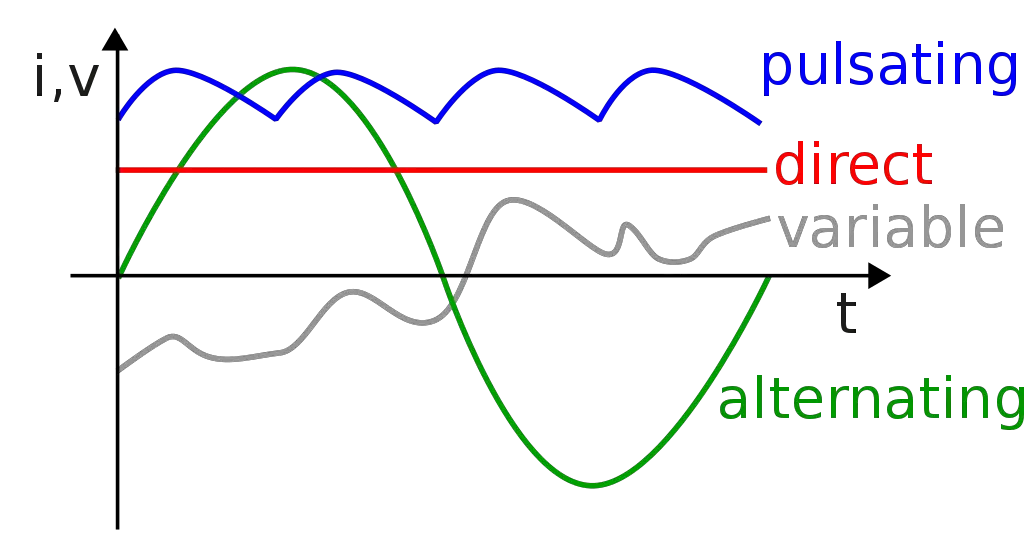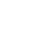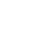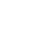# Electric Current

The average person uses a lot of electricity in the many things they do every day. We are used to being able to turn on a light, watch the television, use our mobile devices, and stream music or videos. Because electricity is so much a part of our lives, we should know something about it.If you think about your garden hose you know that in order for it to work you need to turn on the spigot to let the pressure push the water through.

Electrical current operates in a kind of similar way in that it needs something to force the flow through. A “volt” is the measurement of the electrical pressure and is typically supplied by a generator or battery.

When scientists were first discovering the processes of electricity they created symbols for many of the functions.

Since it was still in the early days, they called the used the letter “E” for “Electromotive Force” as a measurement of the force that pushes electricity. As time passed, electricians redefined this as “Volts,” and they use the letter “V.”

### Current: the motion of electricity

We measure electrical current in “amps” (amperes), and this is the measurement of the number of electrons that flow through a conductive material. You might compare it to the amount of water that flows through a garden hose, in a specific amount of time.

In electrical terms, the amps are the amount of electricity that flows through a wire in a specific amount of time. Scientists have given the symbol of “I” for amps.

Knowing the amps in an electrical current charge flow is important, especially when using electronic circuitry.

Current flows through the circuit when voltage is added across two points of a conductor. Think of it as a starting point and then an ending point that lets the flow of electricity through.

### Electron Flow

When electricians and scientists are drawing images (known as schematics) for electrical processes, they will create an electronic circuit picture that shows the flow of electrons.

In most cases they show the flow in the positive charge direction, however, electrons in an electronic circuit are actually flowing in the opposite direction.

### Figuring out current

Electricians and scientists calculate current by measuring the resistance of a circuit when they know the voltage. This could be compared to measuring the amount of water flowing into a bucket using a garden hose in a specific amount of time.

If you kink the hose, less water will be flowing into the bucket in the same measurement of time. If you know the voltage and the resistance, the current can be figured out using the formula:

I=V/R

I = current, V= voltage, and R= resistance.
I = voltage divided by the resistance

The current is used to figure out the power using the following formula:

P = I * V
P = power, I = current, and V = voltage.
power = current times the voltage

### AC/DC (more than just a band)

There are two types of current that are used in almost all of the electronic circuits: AC = alternating current and DC = direct current.

• Direct current is a constant flow of electric charge in one direction. An example of DC is when we use batteries. The electric current is sent in one direction to our smart phones. Many electronics use DC for internal power; sometimes converting AC to DC with a transformer.
• Alternating current is the situation where the electric charge flow is constantly changing its direction. AC is mainly used to transmit power in our power lines. In the U.S., the standard frequency that current alternates in 60 Hertz. Some countries use the standard frequency of 50 Hertz.### Electromagnetism

A magnetic field can be generated by an electric current, and this current is used in such things as electric motors.

• Scientists and electricians create images of current flow with an arrow and in almost all electronic circuits, the current is displayed as flowing towards the ground.
• The tool used to measure a circuit’s current is called an “anmeter.”

### Q&A

What does “AC” stand for?
alternating current

What does “DC” stand for?
direct current

What is a volt?
the measurement of the electrical pressure and is typically supplied by a generator or battery

What are amperes?
the measurement of the number of electrons that flow through a conductive material

•History
•Science
•Geography
•Biography
•US History